# Mathematics Exam Test Quiz Primary 2 Part 1

Last Updated on August 18, 2021 by Quizzes

MATHEMATICS QUIZZES

Choose the correct answer from the options.

Start Quiz

``` ```

``` Results - Last Updated on August 18, 2021 by Quizzes You are Awesome.Related (adsbygoogle = window.adsbygoogle || []).push({}); No related posts. Last Updated on August 18, 2021 by Quizzes Got back to the class.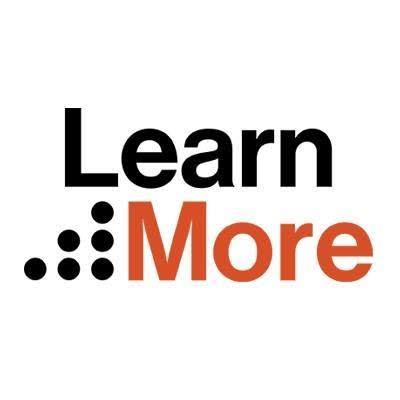Related (adsbygoogle = window.adsbygoogle || []).push({}); No related posts. HD Quiz powered by harmonic design #1. Find the even numbers 3, 2, 7, 4, 11. 4 and 2 4 and 2 3 and 7 3 and 7 3 and 2 3 and 2 7 and 11 7 and 11 #2. Which time is 5 O'clock?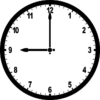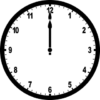#3. Which of these shapes is cylinder?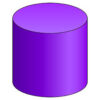#4. Which of these is 5/12?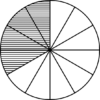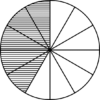#5. Subtract, 67 - 28 = 39 39 40 40 52 52 28 28 (adsbygoogle = window.adsbygoogle || []).push({}); #6. Count in 10s: 10, ___. 30, ___, ___, 60, 70, ___, 90, ___. 20, 40, 50, 80, 100 20, 40, 50, 80, 100 20, 40, 60, 70, 100 20, 40, 60, 70, 100 50, 30, 40, 90, 100 50, 30, 40, 90, 100 60, 70, 80, 90, 100 60, 70, 80, 90, 100 #7. Which time is 12 O'clock?#8. Which time is 9 O'clock?#9. Which of these shapes is cuboid?#10. Find the sum of 6cm, 4cm, 4cm, 6cm. 10 cm 10 cm 8 cm 8 cm 20 cm 20 cm 4 cm 4 cm (adsbygoogle = window.adsbygoogle || []).push({}); #11. Which container contains the most liquid?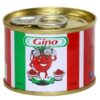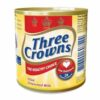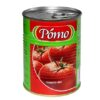#12. Write in words 199. One hundred and ninety One hundred and ninety One hundred and ninety nine One hundred and ninety nine One hundred and nine One hundred and nine One hundred and nineteen One hundred and nineteen #13. Which of these is 4/12?#14. Which container contains the least liquid?#15. Which of these shapes is cube?(adsbygoogle = window.adsbygoogle || []).push({}); #16. 60 minutes make _____ hour. 1 1 2 2 3 3 4 4 #17. Which of the following number shows 2 x 5? 2 + 2 + 2 + 2 2 + 2 + 2 + 2 2 + 2 2 + 2 2 + 2 + 2 + 2 + 2 2 + 2 + 2 + 2 + 2 2 + 2 + 2 2 + 2 + 2 #18. Mark the smallest number 50, 20, 50, 80. 80 80 60 60 50 50 20 20 #19. Which time is 3 O'clock?#20. Which of these is 2/12?(adsbygoogle = window.adsbygoogle || []).push({}); finish ```
``` ```